Anzeige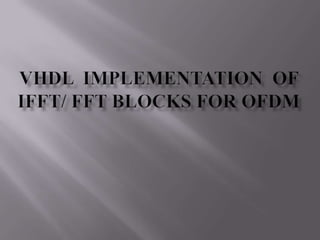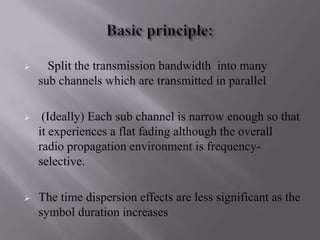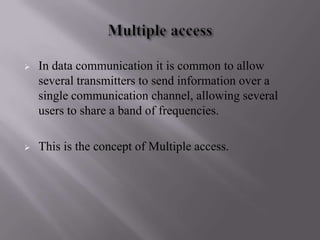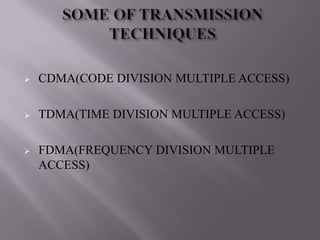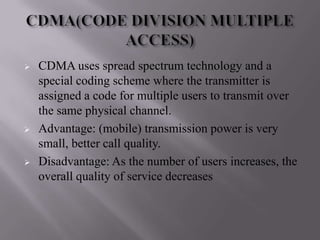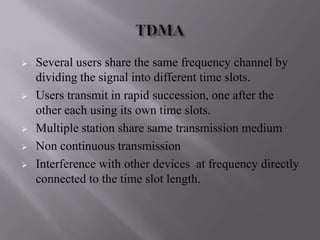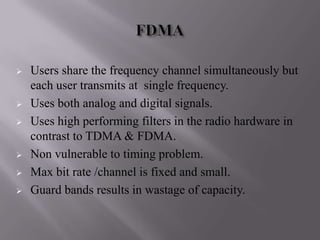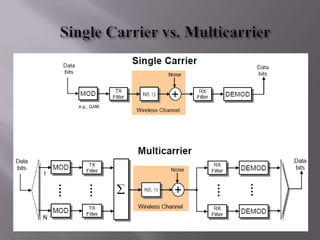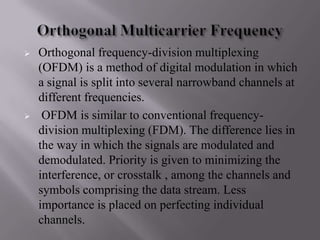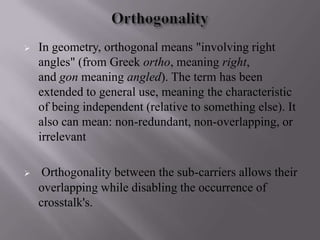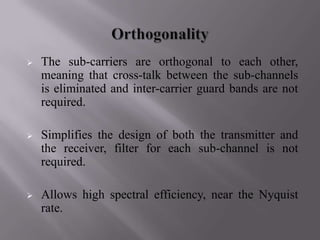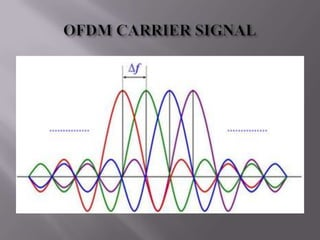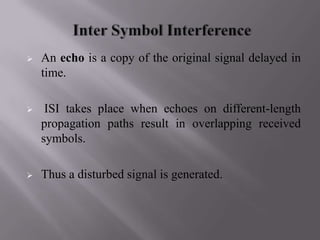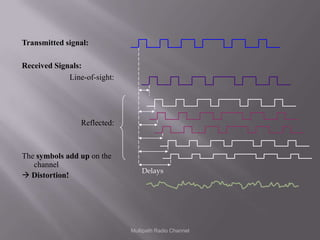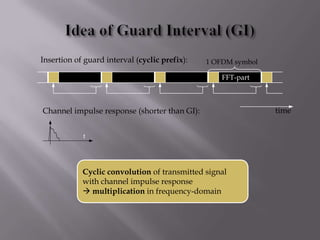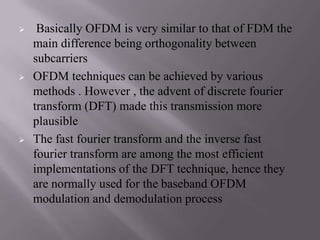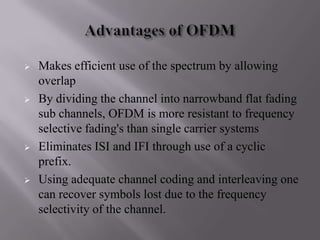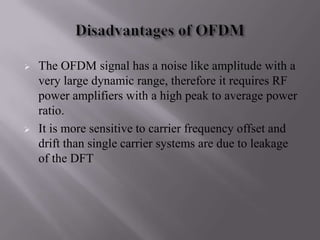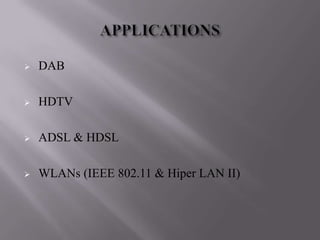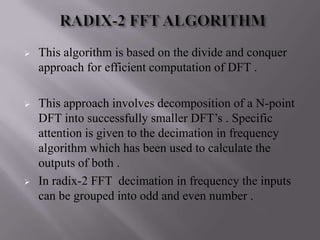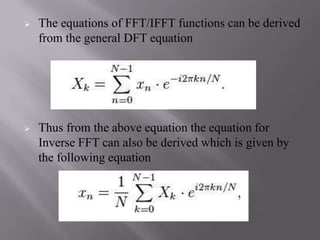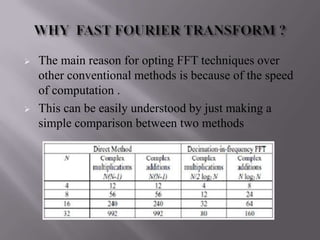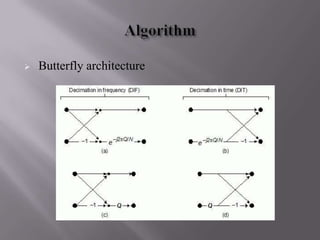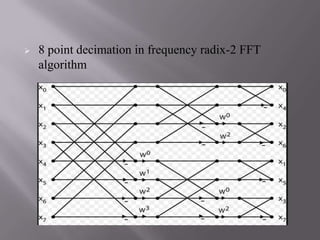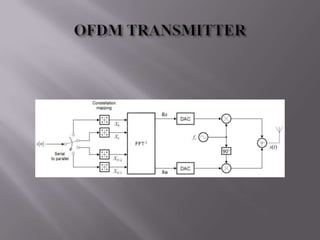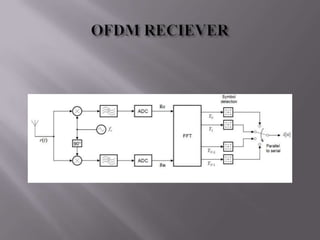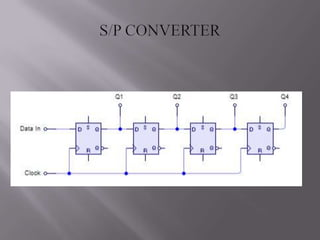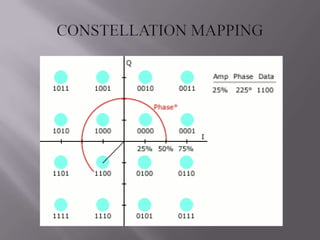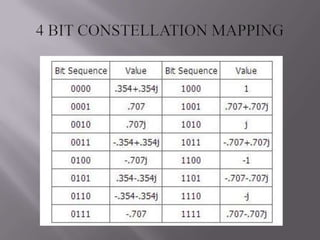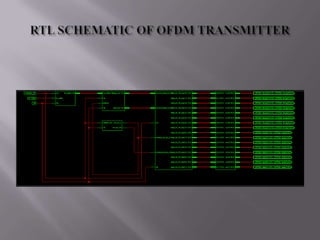1 von 32
Anzeige

### Vhdl implementation of ofdm transmitter

1.  Split the transmission bandwidth into many sub channels which are transmitted in parallel  (Ideally) Each sub channel is narrow enough so that it experiences a flat fading although the overall radio propagation environment is frequency- selective.  The time dispersion effects are less significant as the symbol duration increases
2.  In data communication it is common to allow several transmitters to send information over a single communication channel, allowing several users to share a band of frequencies.  This is the concept of Multiple access.
3.  CDMA(CODE DIVISION MULTIPLE ACCESS)  TDMA(TIME DIVISION MULTIPLE ACCESS)  FDMA(FREQUENCY DIVISION MULTIPLE ACCESS)
4.  CDMA uses spread spectrum technology and a special coding scheme where the transmitter is assigned a code for multiple users to transmit over the same physical channel.  Advantage: (mobile) transmission power is very small, better call quality.  Disadvantage: As the number of users increases, the overall quality of service decreases
5.  Several users share the same frequency channel by dividing the signal into different time slots.  Users transmit in rapid succession, one after the other each using its own time slots.  Multiple station share same transmission medium  Non continuous transmission  Interference with other devices at frequency directly connected to the time slot length.
6.  Users share the frequency channel simultaneously but each user transmits at single frequency.  Uses both analog and digital signals.  Uses high performing filters in the radio hardware in contrast to TDMA & FDMA.  Non vulnerable to timing problem.  Max bit rate /channel is fixed and small.  Guard bands results in wastage of capacity.
7.  Orthogonal frequency-division multiplexing (OFDM) is a method of digital modulation in which a signal is split into several narrowband channels at different frequencies.  OFDM is similar to conventional frequency- division multiplexing (FDM). The difference lies in the way in which the signals are modulated and demodulated. Priority is given to minimizing the interference, or crosstalk , among the channels and symbols comprising the data stream. Less importance is placed on perfecting individual channels.
8.  In geometry, orthogonal means "involving right angles" (from Greek ortho, meaning right, and gon meaning angled). The term has been extended to general use, meaning the characteristic of being independent (relative to something else). It also can mean: non-redundant, non-overlapping, or irrelevant  Orthogonality between the sub-carriers allows their overlapping while disabling the occurrence of crosstalk's.
9.  The sub-carriers are orthogonal to each other, meaning that cross-talk between the sub-channels is eliminated and inter-carrier guard bands are not required.  Simplifies the design of both the transmitter and the receiver, filter for each sub-channel is not required.  Allows high spectral efficiency, near the Nyquist rate.
10.  An echo is a copy of the original signal delayed in time.  ISI takes place when echoes on different-length propagation paths result in overlapping received symbols.  Thus a disturbed signal is generated.
11. Multipath Radio Channel Transmitted signal: Received Signals: Line-of-sight: Reflected: The symbols add up on the channel  Distortion! Delays
12.  It is advantageous to transmit a number of low-rate streams in parallel instead of a single high-rate stream.  Since the duration of each symbol is long, it is feasible to insert a guard interval between the OFDM symbols,  Thus eliminating the intersymbol interference.
13. FFT-part time Insertion of guard interval (cyclic prefix): Channel impulse response (shorter than GI): t 1 OFDM symbol Cyclic convolution of transmitted signal with channel impulse response  multiplication in frequency-domain
14.  Basically OFDM is very similar to that of FDM the main difference being orthogonality between subcarriers  OFDM techniques can be achieved by various methods . However , the advent of discrete fourier transform (DFT) made this transmission more plausible  The fast fourier transform and the inverse fast fourier transform are among the most efficient implementations of the DFT technique, hence they are normally used for the baseband OFDM modulation and demodulation process
15.  Makes efficient use of the spectrum by allowing overlap  By dividing the channel into narrowband flat fading sub channels, OFDM is more resistant to frequency selective fading's than single carrier systems  Eliminates ISI and IFI through use of a cyclic prefix.  Using adequate channel coding and interleaving one can recover symbols lost due to the frequency selectivity of the channel.
16.  The OFDM signal has a noise like amplitude with a very large dynamic range, therefore it requires RF power amplifiers with a high peak to average power ratio.  It is more sensitive to carrier frequency offset and drift than single carrier systems are due to leakage of the DFT
17.  DAB  HDTV  ADSL & HDSL  WLANs (IEEE 802.11 & Hiper LAN II)
18.  This algorithm is based on the divide and conquer approach for efficient computation of DFT .  This approach involves decomposition of a N-point DFT into successfully smaller DFT’s . Specific attention is given to the decimation in frequency algorithm which has been used to calculate the outputs of both .  In radix-2 FFT decimation in frequency the inputs can be grouped into odd and even number .
19.  The equations of FFT/IFFT functions can be derived from the general DFT equation  Thus from the above equation the equation for Inverse FFT can also be derived which is given by the following equation
20.  The main reason for opting FFT techniques over other conventional methods is because of the speed of computation .  This can be easily understood by just making a simple comparison between two methods
21.  Butterfly architecture
22.  8 point decimation in frequency radix-2 FFT algorithm
Anzeige